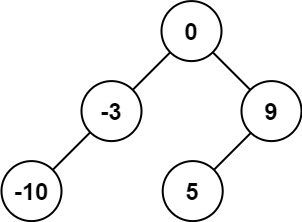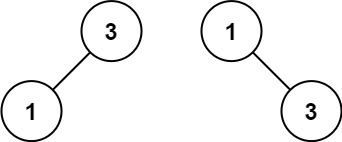# 108. Convert Sorted Array to Binary Search Tree

Easy
Given an integer array `nums` where the elements are sorted in ascending order, convert it to a height-balanced binary search tree.
A height-balanced binary tree is a binary tree in which the depth of the two subtrees of every node never differs by more than one.
Example 1:Input: nums = [-10,-3,0,5,9]
Output:
[0,-3,9,-10,null,5]
Explanation:
[0,-10,5,null,-3,null,9] is also accepted:
Example 2:Input: nums = [1,3]
Output:
[3,1]
Explanation:
[1,null,3] and [3,1] are both height-balanced BSTs.
Constraints:
• `1 <= nums.length <= 104`
• `-104 <= nums[i] <= 104`
• `nums` is sorted in a strictly increasing order.

### 解題

Runtime: 0 ms, faster than 100.00%
Memory Usage: 3.5 MB, less than 76.66%
/**
* Definition for a binary tree node.
* type TreeNode struct {
* Val int
* Left *TreeNode
* Right *TreeNode
* }
*/
func sortedArrayToBST(nums []int) *TreeNode {
var create func(int, int) *TreeNode
create = func(start int, end int) *TreeNode {
if start > end { return nil }
mid := (start + end)/2
root := &TreeNode{ Val: nums[mid] }
root.Left = create(start, mid-1)
root.Right = create(mid+1, end)
return root
}
return create(0, len(nums)-1)
}

func sortedArrayToBST(nums []int) *TreeNode {
if len(nums) == 0 {
return nil
}
return &TreeNode{
Val: nums[len(nums)/2],
Left: sortedArrayToBST(nums[:len(nums)/2]),
Right: sortedArrayToBST(nums[len(nums)/2 + 1:]),
}
}